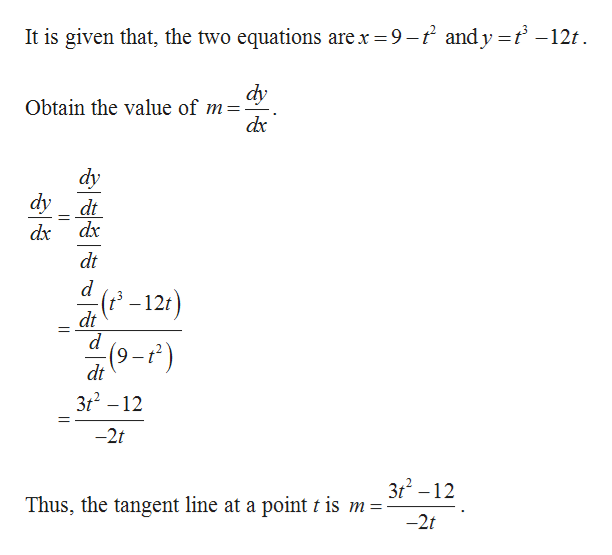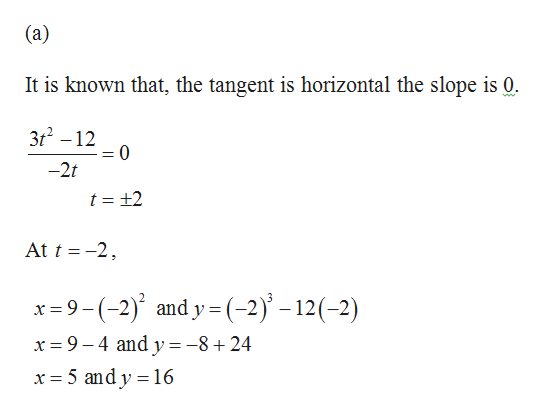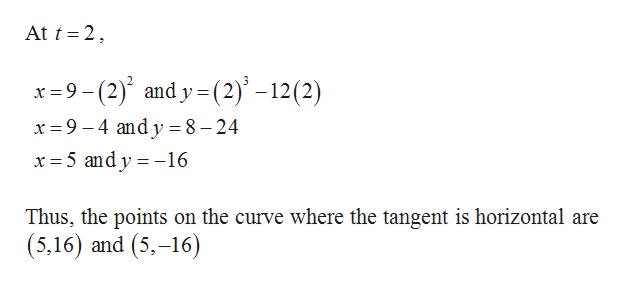# Use the given parameters to answer the following questions. If you have a graphing device, graph the curve to check your work.x = 9 - t2y = t3 - 12t(a) Find the points on the curve where the tangent is horizontal.(b) Find the points on the curve where the tangent is vertical.

Question
3 views

Use the given parameters to answer the following questions. If you have a graphing device, graph the curve to check your work.
x = 9 - t2
y = t3 - 12t

(a) Find the points on the curve where the tangent is horizontal.

(b) Find the points on the curve where the tangent is vertical.

check_circle

Step 1help_outlineImage TranscriptioncloseIt is given that, the two equations are x 9-2 andy = t -12t dy Obtain the value of m dx dy dy dt dx dx dt d (2-12t) dt d (9-r) dt 3t2 -12 -2t 3t2-12 Thus, the tangent line at a point t is m = -2t fullscreen
Step 2help_outlineImage Transcriptionclose(a) It is known that, the tangent is horizontal the slope is 0. 3t2 -12 0 -2t t = +2 At t -2 x =9-(-2) and y-(-2-12(-2) x 9-4 and y=-8+24 x 5 and y 16 fullscreen
Step 3help_outlineImage TranscriptioncloseAt t 2 x9-(2) and y (2)-12(2) x 9-4 andy= 8 - 24 x 5 and y16 Thus, the points on the curve where the tangent is horizontal are (5,16) and (5,-16) fullscreen

### Want to see the full answer?

See Solution

#### Want to see this answer and more?

Solutions are written by subject experts who are available 24/7. Questions are typically answered within 1 hour.*

See Solution
*Response times may vary by subject and question.
Tagged in

### Other